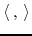Madagascar Programming Reference ManualNext: Implementation of operators Up: Introduction Previous: Adjoint operators

## The dot-product test

The dot-product test is a valuable checkpoint, which can tell us whether the implementation of the adjoint operator is wrong (however it cannot guarantee that it is indeed correct). The concept is the following: Assuming that we have coded an operatorand its adjoint. Then for any two vectors or functionsand,(1)

wheredenotes the dot product. Remember that the dot product of two functionsiswhile the dot product of two vectorsandis. Notice that for vectors eq. (1) becomeswhich is obviously true. The lhs of eq. (1) is computed using, while the rhs is computed using the adjoint. For the dot-product test, one just needs to load the vectors x and y with random numbers and perform the two computations. If the two results are not equal (within machine precision), then the computation of eitheroris erroneous. Note that truncation errors have identical effects on both operators, so the two results should be almost equal. The dot-product test (for real operators only) is implemented by [sec:sf_dot_test]sf_dot_test.Madagascar Programming Reference ManualNext: Implementation of operators Up: Introduction Previous: Adjoint operators

2011-07-02